# SSC JE Electrical Important MCQ Part 13

SSC JE Electrical Important MCQ PDF Part 13

1.The value of vₒ and i in the circuit shown in Fig is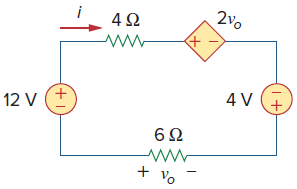A. vₒ=-24,i=-4

B. vₒ=48,i=-8

C. vₒ=24,i=-8

D. vₒ=-48,i=4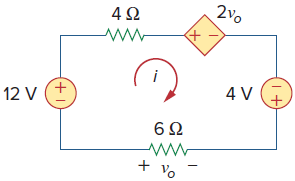Apply KVL around the loop as shown in Fig. above
−12 + 4i + 2vₒ − 4 + 6i = 0  —–(1)
Applying Ohm’s law to the 6-Ω resistor gives
vₒ = −6i
Substituting vₒ into Eq. (1)
−16 + 10i − 12i = 0 ⇒ i = −8 A
And vₒ = −6i⇒vₒ =-6×-8= 48 V

2.Circuit shown in fig. is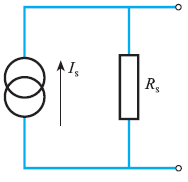A. Thévenin’s equivalent circuit

B. Norton’s equivalent circuit

C. Superposition equivalent circuit

D. Dependent current source equivalent circuit

Answer  : B (Norton’s theorem states that any two terminal linear network or circuit can be represented with an equivalent network or circuit, which consists of a current source in parallel with a resistor)

3.Symbol shown in fig. is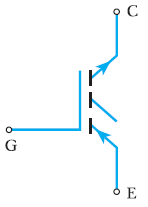A. GTO

B. BJT

C. MOSFET

D. IGBT

Answer  : D (An insulated-gate bipolar transistor (IGBT) is a three-terminal power semiconductor device primarily used as an electronic switch )

4.Value of Ceq in below figure is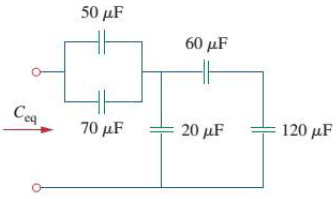A. 20μF

B. 60μF

C. 30μF

D. 40μF

Equivalent C of series 60 μF & 120 μF=60*120/180=40 μF

This 40 μF is in parallel with 20 μF=40+20=60 μF

And 50 μF and 70 μF in parallel=50+70=120 μF

So Ceq=60*120/180=40 μF

5.In the circuit in Fig. R = 2 &, L = 1 mH, and C = 0.4 μF.Then resonant frequency is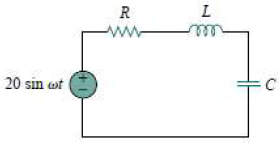6.Value of Rth across a-b terminal is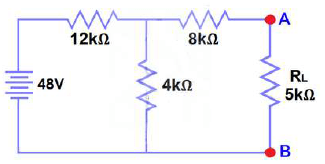A. 11 kΩ

B. 4 kΩ

C. 12 kΩ

D. 16 kΩ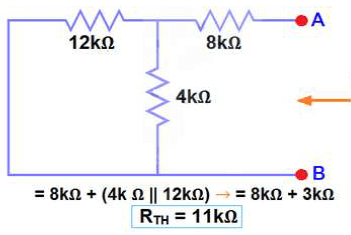7.Fault shown in fig. is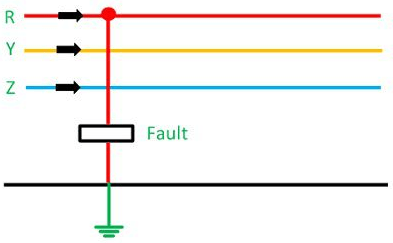A. Single Line-to-ground (L – G) Fault

B. Line-to-Line Fault (L – L)

C. Double Line-to-ground (L – L – G) Fault

D. Three-phase line to ground (L – L – L – G) fault

Answer  : A (L – G Fault-The single line of ground fault occurs when one conductor falls to the ground or contact the neutral conductor)

8.The terminal voltage of a generator which develops an e.m.f. of 200 V and has an armature current of 30 A on load. Assume the armature resistance is 0.30 Ω

A. 209 volts

B. 191 volts

C. 220 volts

D. 210 volts

9.The normal value of adhension friction is
A. 0.12

B. 0.25

C. 0.40

D. 0.75

10.The highest illumination is required

A. For office work

B. For mounting of wrist watches

C.For sorting work in stores

D. For painting work

11.Desirable property of resistance heating element materials is

A. high resistivity

B. high melting point

C. low temperature coefficient

D. all of these

12.The design of insulation for systems above 400 kV, is based upon

A. Lightning over voltage

B. Switching surges

C. System voltage level

13.In order to have lower cost of electrical energy generation

A. The load factor and diversity factor should be low

B. The load factor should be low but diversity factor should be high

C. The load factor should be high but diversity factor low

D.The load factor and diversity factors should be high

Answer  : D (If load factor and diversity factors are high, maximum demand will be less, it will reduce the machine rating and lower cost of power generation is possible)

14.Quantities having the unit “lux” is

A. Utilization factor

B. luminous flux

C. luminous intensity

D. illumination

Answer  : D (The lux is the SI derived unit of illuminaTIONnce, measuring luminous flux per unit area)

15.Which of the following is of high importance in case of induction heating

A. Voltage

B. Current

C. Frequency

D. All of these

16.When t is the thickness of the sheet, the tip diameter for spot welding is usually

A. 2t

B. t

C. √t

D. 1/𝑡

Answer  : C (Tip diameter that are affected by the thickness of the worksheet is 5√t)

17.TIG welding is

A. Temperature insulated gas welding

B. Tungsten inert gas welding

C. Thermally induced gas welding

D. Thorium iodine gas welding

Answer  : B (Tungsten Inert Gas (TIG) welding, also known as Gas Tungsten Arc Welding (GTAW) is an arc welding process that produces the weld with a non-consumable tungsten electrode)

A. Same on a.c. and d.c. traction systems

B. High in case of d.c. traction and low in a.c. traction

C. High in case of a.c. traction and low in d.c. traction

D. None of these

19.Earthing is used as the return conductor for

A.Telephone lines

B. Telegraph lines

C. Traction work

D. All of these

20.Mixture preferred for filling around the earth for electrode effective earthing is

A. bone-meat mixture

B. coal-salt mixture

C. saw-dust sand mixture

D. lime-sand mixture